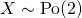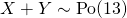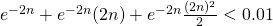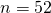# 2014 A-level H2 Mathematics (9740) Paper 2 Question 11 Suggested SolutionsAll solutions here are SUGGESTED. Mr. Teng will hold no liability for any errors. Comments are entirely personal opinions.

(i)
Let X and Y be the number of originals and prints sold in a week, respectively.(a)(b)(ii) Let W be the number of originals sold inweeks.Using GC, smallest possible n = 5.

(iii) Sinceis large, by Central Limit Theorem,approximately.(iv) Firstly the mean number of paintings sold per week may not be constant due to seasonal factors. Secondly, the sales might not be independent as a buyer might have preferential taste for particular artists.

For (ii), students are expected to draw out the table to illustrate the choice of n. For (iii), students can either use CLT or approximate by normal distribution but do remember to do continuity correction then. I did CLT here since I haven’t done CLT for the entire paper while I did approximation in Question 7.If students do by approximation of normal distribution, they should expect the answer to be 0.816.

Not readable? Change text.